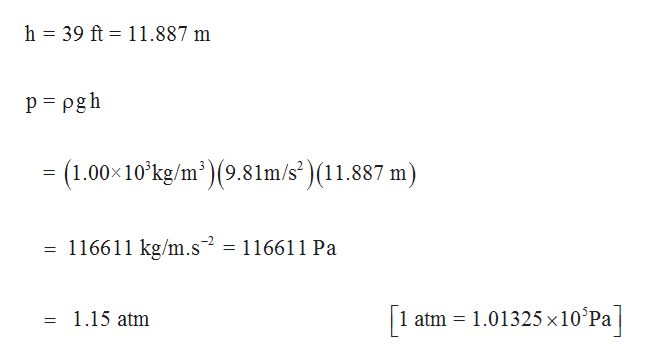# What pressure, in atmospheres, is exerted on the body of a diver if she is 39 ft below the surface of the water when atmospheric pressure at the surface is 720 mmHg ? Assume that the density of the water is 1.00g/cm3=1.00×103kg/m3. The gravitational constant is 9.81m/s2, and 1Pa=1kg/m−s2.

Question
6 views

What pressure, in atmospheres, is exerted on the body of a diver if she is 39 ft below the surface of the water when atmospheric pressure at the surface is 720 mmHg ? Assume that the density of the water is 1.00g/cm3=1.00×103kg/m3. The gravitational constant is 9.81m/s2, and 1Pa=1kg/m−s2.

check_circle

Step 1

Pressure exerted by water is ...help_outlineImage Transcriptioncloseh 39 ft 11.887 m p pgh (1.00 10 kg/m)(9.81m/s')(11.887 m) 116611 kg/m.s2 116611 Pa atm 1.01325 x10°Pa 1.15 atm fullscreen

### Want to see the full answer?

See Solution

#### Want to see this answer and more?

Solutions are written by subject experts who are available 24/7. Questions are typically answered within 1 hour.*

See Solution
*Response times may vary by subject and question.
Tagged in

### Gas laws